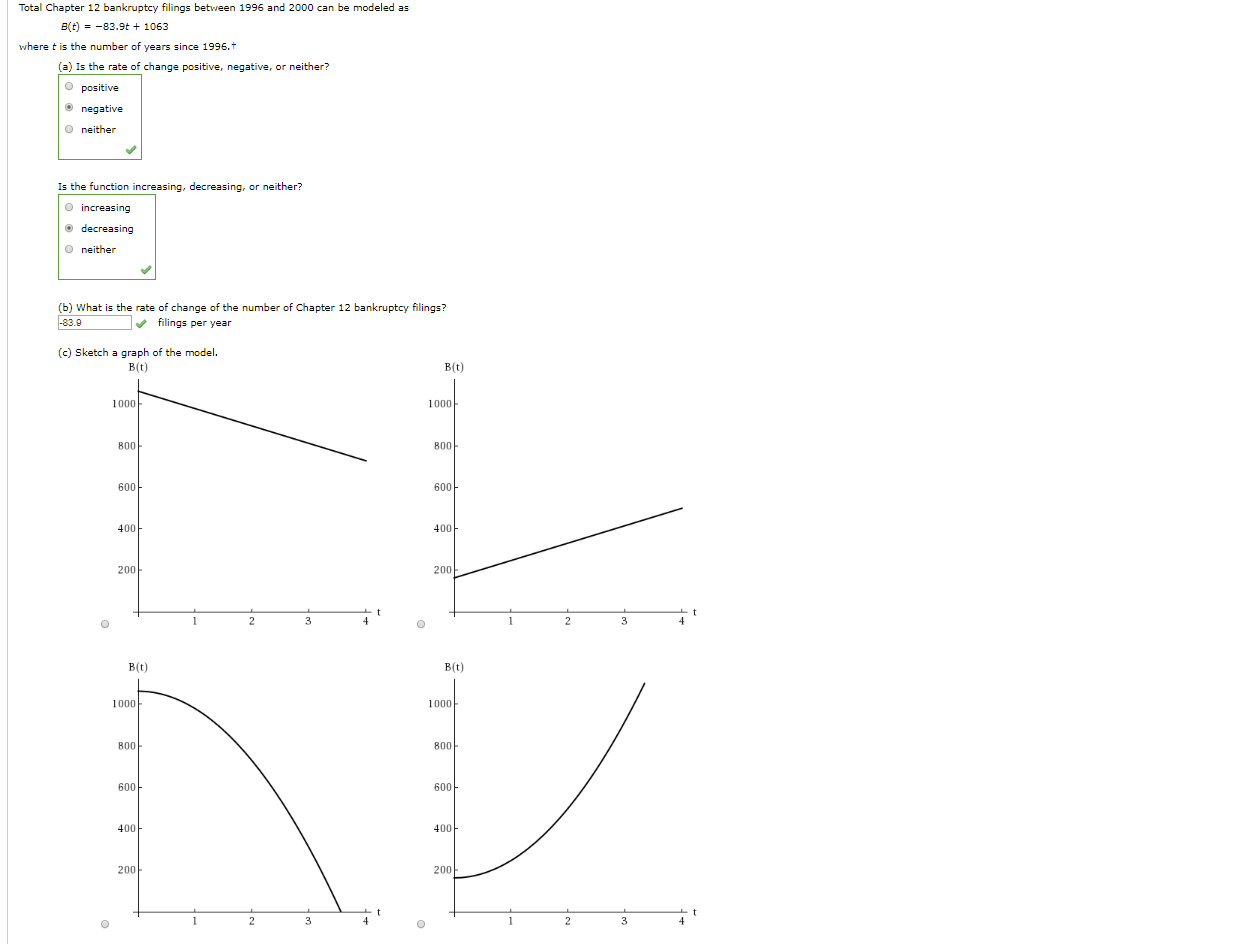# Total Chapter 12 bankruptcy filings between 1996 and 2000 can be modeled asB(t)-83.9t1063where t is the number of years since 1996.t(a) Is the rate of change positive, negative, or neither?O positivenegativeO neitherIs the function increasing, decreasing, or neither?OincreasingdecreasingO neither(b) What is the rate of change of the number of Chapter 12 bankruptcy filings?-83.9filings per year(c) Sketch a graph of the modelB (t)B(t)10001000800800600600400400200200tt234234B(t)B(t)10001000800800600600400400200200234234C

Question
68 views

What is the slope of the graph?

(d) Find the output for which the input is three.

Explain its significance in context. (Hint: Round to the context.)

In the year_________________, there were______________Chapter 12 bankruptcy filings.help_outlineImage TranscriptioncloseTotal Chapter 12 bankruptcy filings between 1996 and 2000 can be modeled as B(t)-83.9t1063 where t is the number of years since 1996.t (a) Is the rate of change positive, negative, or neither? O positive negative O neither Is the function increasing, decreasing, or neither? Oincreasing decreasing O neither (b) What is the rate of change of the number of Chapter 12 bankruptcy filings? -83.9 filings per year (c) Sketch a graph of the model B (t) B(t) 1000 1000 800 800 600 600 400 400 200 200 t t 2 3 4 2 3 4 B(t) B(t) 1000 1000 800 800 600 600 400 400 200 200 2 3 4 2 3 4 C fullscreen
check_circle

Step 1

For a standard equation of straight line: y = mx + c; the slope of the line is represented by "m".

Step 2

In this case, the equation of the staright line is: B(t) = - 83.9t + 1,063

Let's compare this with y = mx + c

By observation and comprison, we can figure out that slope of the line = m = - 83.9

Hence, the slope of the graph is - 83.9

Step 3

If the input is 3, we must assign, t = 3 in the equation above. The output = B(t = 3) = - 83.9 x 3 + 1,063 = 811.30

However, please note that he output is number of cases and hence can't be fractional. It has to be an integral...

### Want to see the full answer?

See Solution

#### Want to see this answer and more?

Solutions are written by subject experts who are available 24/7. Questions are typically answered within 1 hour.*

See Solution
*Response times may vary by subject and question.
Tagged in

### Functions